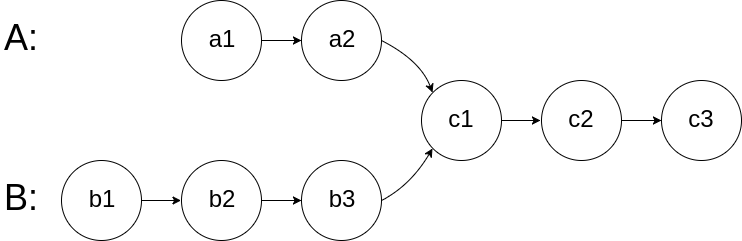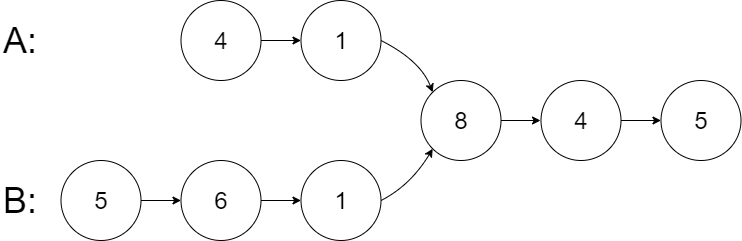New update is available. Click here to update.

# Intersection of Two Linked Lists

Contributed by
Arshit Babariya
Last Updated: 23 Feb, 2023
Easy0/40
Avg time to solve 25 mins
Success Rate 73 %Share139 upvotes

## Problem Statement

Suggest Edit

#### For example:-

``````The given Linked Lists, where a1, a2, c1 is the first linked list, b1, b2, b3, c1 is the second linked list, and c1, c2, c3 is the third linked list which are merging at node c1.
``````Detailed explanation ( Input/output format, Notes, Images )##### Constraints :
``````0 <= N <= 10^5
0 <= M <= 10^5
0 <= K <= 10^5.
-10^9 <= data <= 10^9 and data != -1

Time Limit: 1 sec
``````
##### Sample Input 1 :
``````4 1 8 -1
5 6 1 8 -1
4 5 -1
``````
##### Sample Output 1 :
``````8
``````
##### Explanation For Sample Input 1:
``````As shown in the diagram the node with data is 8 at which merging starts
``````##### Sample Input 2 :
``````1 9 1 2 -1
3 2 -1
4 -1
``````
##### Sample Output 2 :
``````2
``````
##### Sample Input 3 :
``````2 6 4 -1
1 5 -1
-1
``````
##### Sample Output 3 :
``````-1
``````AutoConsole